## Dissection

Any two rectilinear figures with equal Area can be dissected into a finite number of pieces to form each other. This is the Wallace-Bolyai-Gerwein Theorem. For minimal dissections of a Triangle, Pentagon, and Octagon into a Square, see Stewart (1987, pp. 169-170) and Ball and Coxeter (1987, pp. 89-91). The Triangle to Square dissection (Haberdasher's Problem) is particularly interesting because it can be built from hinged pieces which can be folded and unfolded to yield the two shapes (Gardner 1961; Stewart 1987, p. 169; Pappas 1989).Laczkovich (1988) proved that the Circle can be squared in a finite number of dissections (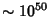). Furthermore, any shape whose boundary is composed of smoothly curving pieces can be dissected into a Square.

The situation becomes considerably more difficult moving from 2-D to 3-D. In general, a Polyhedron cannot be dissected into other Polyhedra of a specified type. A Cube can be dissected into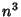Cubes, whereis any Integer. In 1900, Dehn proved that not every Prism cannot be dissected into a Tetrahedron (Lenhard 1962, Ball and Coxeter 1987). The third of Hilbert's Problems asks for the determination of two Tetrahedra which cannot be decomposed into congruent Tetrahedra directly or by adjoining congruent Tetrahedra. Max Dehn showed this could not be done in 1902, and W. F. Kagon obtained the same result independently in 1903. A quantity growing out of Dehn's work which can be used to analyze the possibility of performing a given solid dissection is the Dehn Invariant.

The table below is an updated version of the one given in Gardner (1991, p. 50). Many of the improvements are due to G. Theobald (Frederickson 1997). The minimum number of pieces known to dissect a regular-gon (whereis a number in the first column) into a-gon (whereis a number is the bottom row) is read off by the intersection of the corresponding row and column. In the table,denotes a regular-gon, GR a Golden Rectangle, GC a Greek Cross, LC a Latin Cross, MC a Maltese Cross, SW a Swastika,a five-point star (solid Pentagram),a six-point star (i.e., Hexagram or solid Star of David), andthe solid Octagram.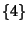4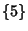6 65 5 78 7 9 87 5 9 8 118 9 12 11 14 137 7 10 9 11 10 13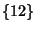8 6 10 6 11 10 14 12 GR 4 3 6 5 7 6 9 6 7 GC 5 4 7 7 9 9 12 10 6 5 LC 5 5 8 6 8 8 11 10 7 5 7 MC 7 14 8 SW 6 12 8 97 7 9 9 11 10 14 6 12 7 10 105 5 8 6 9 8 11 9 9 5 8 8 118 8 9 9 12 6 13 12 12 7 10 11 13 10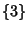GR GC LC MC SWThe best-known dissections of one regular convex-gon into another are shown for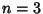, 4, 5, 6, 7, 8, 9, 10, and 12 in the following illustrations due to Theobald.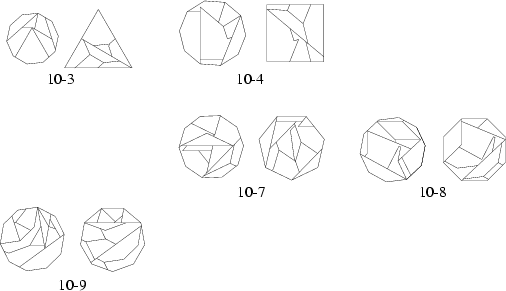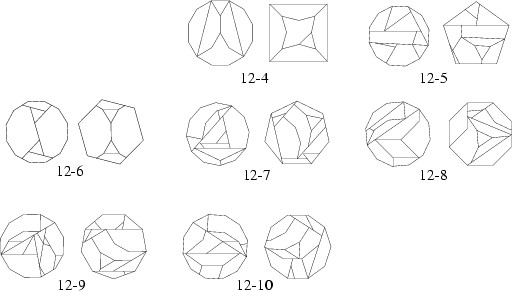The best-known dissections of regular concave polygons are illustrated below for,, and(Theobald).The best-known dissections of various crosses are illustrated below (Theobald).The best-known dissections of the Golden Rectangle are illustrated below (Theobald).See also Banach-Tarski Paradox, Cundy and Rollett's Egg, Decagon, Dehn Invariant, Diabolical Cube, Dissection Puzzles, Dodecagon, Ehrhart Polynomial, Equidecomposable, Equilateral Triangle, Golden Rectangle, Heptagon, Hexagon, Hexagram, Hilbert's Problems, Latin Cross, Maltese Cross, Nonagon, Octagon, Octagram, Pentagon, Pentagram, Polyhedron Dissection, Pythagorean Square Puzzle, Pythagorean Theorem, Rep-Tile, Soma Cube, Square, Star of Lakshmi, Swastika, T-Puzzle, Tangram, Wallace-Bolyai-Gerwein Theorem

References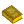Dissections

Ball, W. W. R. and Coxeter, H. S. M. Mathematical Recreations and Essays, 13th ed. New York: Dover, pp. 87-94, 1987.

Coffin, S. T. The Puzzling World of Polyhedral Dissections. New York: Oxford University Press, 1990.

Cundy, H. and Rollett, A. Ch. 2 in Mathematical Models, 3rd ed. Stradbroke, England: Tarquin Pub., 1989.

Eppstein, D. Dissection.'' http://www.ics.uci.edu/~eppstein/junkyard/dissect.html.

Eppstein, D. Dissection Tiling.'' http://www.ics.uci.edu/~eppstein/junkyard/distile/.

Eriksson, K. Splitting a Polygon into Two Congruent Pieces.'' Amer. Math. Monthly 103, 393-400, 1996.

Frederickson, G. Dissections: Plane and Fancy. New York: Cambridge University Press, 1997.

Gardner, M. The Second Scientific American Book of Mathematical Puzzles & Diversions: A New Selection. New York: Simon and Schuster, 1961.

Gardner, M. Paper Cutting.'' Ch. 5 in Martin Gardner's New Mathematical Diversions from Scientific American. New York: Simon and Schuster, 1966.

Gardner, M. The Unexpected Hanging and Other Mathematical Diversions. Chicago, IL: Chicago University Press, 1991.

Hunter, J. A. H. and Madachy, J. S. Mathematical Diversions. New York: Dover, pp. 65-67, 1975.

Kraitchik, M. Dissection of Plane Figures.'' §8.1 in Mathematical Recreations. New York: W. W. Norton, pp. 193-198, 1942.

Laczkovich, M. Von Neumann's Paradox with Translation.'' Fund. Math. 131, 1-12, 1988.

Lenhard, H.-C. Über fünf neue Tetraeder, die einem Würfel äquivalent sind.'' Elemente Math. 17, 108-109, 1962.

Lindgren, H. Geometric Dissections.'' Austral. Math. Teacher 7, 7-10, 1951.

Lindgren, H. Geometric Dissections.'' Austral. Math. Teacher 9, 17-21, 1953.

Lindgren, H. Going One Better in Geometric Dissections.'' Math. Gaz. 45, 94-97, 1961.

Lindgren, H. Recreational Problems in Geometric Dissection and How to Solve Them. New York: Dover, 1972.

Madachy, J. S. Geometric Dissection.'' Ch. 1 in Madachy's Mathematical Recreations. New York: Dover, pp. 15-33, 1979.

Pappas, T. A Triangle to a Square.'' The Joy of Mathematics. San Carlos, CA: Wide World Publ./Tetra, pp. 9 and 230, 1989.

Stewart, I. The Problems of Mathematics, 2nd ed. Oxford, England: Oxford University Press, 1987.